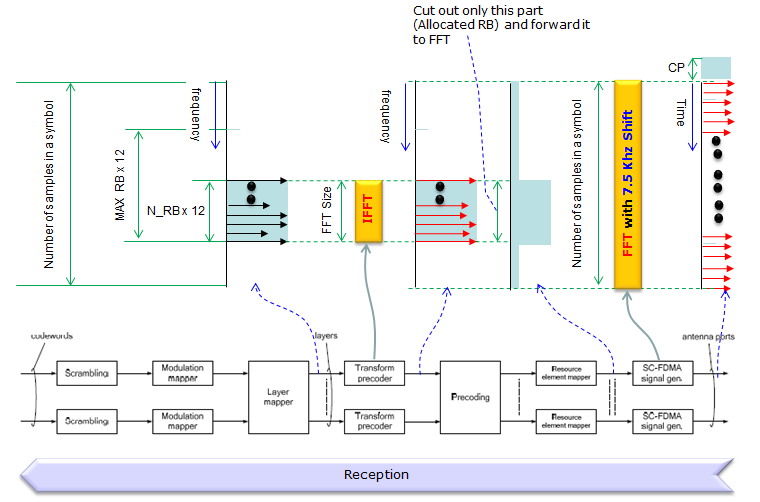LTE Quick Reference RE Map to Antenna / Antenna to RE Map   This is basically for OFDM/SC-FDM at high level. But I tried to describe this in such as way that is helpful for you to program this process (Actually this is based on my experience of coding this part in matlab). However, I will not write on this procedure in full details with each specific numbers and data since it is too much for the single page. For further details, I will put the link to various different pages which would give you a specific examples and details.   < Downlink : RE Map to Antenna >   In this section, I will describe the process highligted as shown below. May look simple single step, but it is pretty complicated process and confusing.. it would be very hard in very detail unless you try to program (code) this process. In this page, I will describe the process in the unit of a slot. If you think you understand overall logics explained here, refer to the example in OFDM page (the example in the page is for WLAN, not for LTE.. but overall logic is almost same). If you want LTE specific example for this process, refer to following two pages.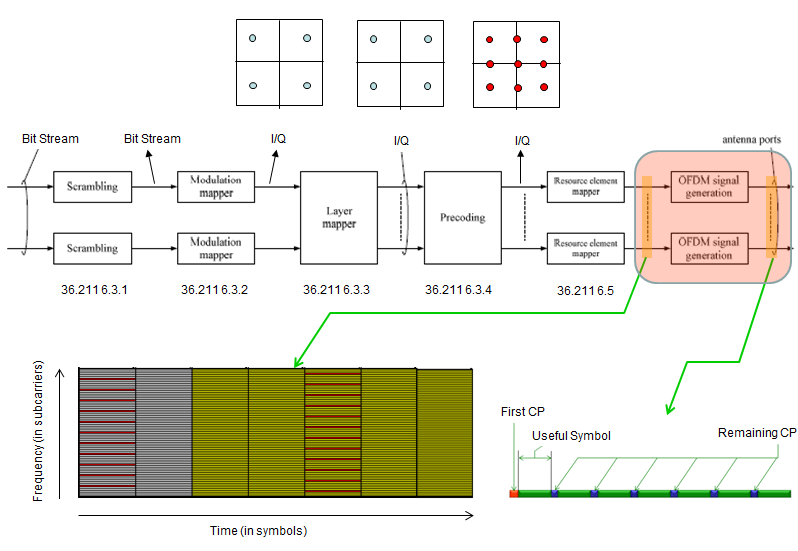Adding Zero Pads : After you get the resource element map with all the data filled in, add a trails of zeros (Zero Pad) on both sides of each column (OFDM Symbol) of the resource map as shown below. Now your question would be, how many zeros I need to add ? What would be the number of data points for each section ?. The answer is different depending on System Bandwidth. For this, refer to page : Physical Layer Parameters - FDD, Downlink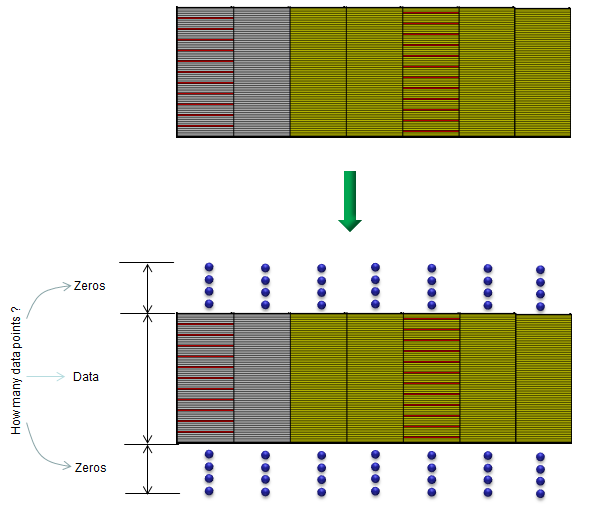Doing IFFT: Do IFFT for each OFDM Symbol and you will get the time domain data for each of OFDM Symbol. What would be the number of data points in each of the time domain OFDM Symbol ? The answer is different depending on System Bandwidth. For this, refer to page : Physical Layer Parameters - FDD, DownlinkAdding Cyclic Prefix : Now add Cyclick Prefix for each of the symbol. How many samples in each of the cyclic prefix ? The answer gets different whether it is the first symbol or non-first symbol and depends on the system bandwidth. For this, refer to page : Physical Layer Parameters - FDD, Downlink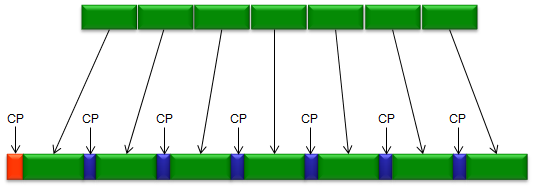D/A Converter/Up Converter : The last step is to convert the symbol data to analog and unconvert it to the carrier frequency and transmitted to Antenna. I think I oversimplified this step and this step would required complicated/sophisticated hardware design, but this is out of the scope of this page.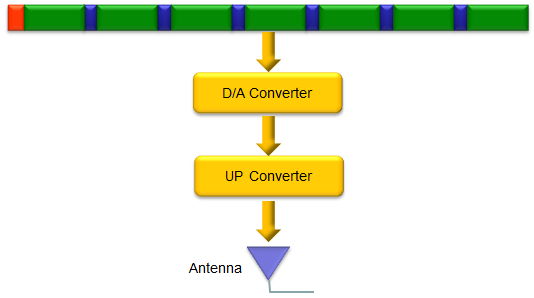< Downlink :  Antenna to RE Map >   This is about receiving the OFDMA signal and demodulate it and construct RE Map from the demodulated bit stream. In reality, demodulation process is much more complicated than the modulation because you would require a lot of channel estimation and compenstion (Equalization), and finding synchronization. However, I assume that we are in a ideal channel and no need for this kind of compensation or synchronization. Once you understand the overall logic of this process, try your understanding with a real example : LTE DL(OFDM) Demodulation.   Down convert and Sampling (A/D Converter) : The first step is to downconvert the signal received by the antenna, downconvert it and sample it with A/D Converter. Assuming that everything is under ideal condition, the most important this to determined the proper sampling rate. Ideally, you can set any sampling rate as far as it is above the Nyquist Criterial, but it would help a lot in following steps if you sample it as specified in  Physical Layer Parameters - FDD, Downlink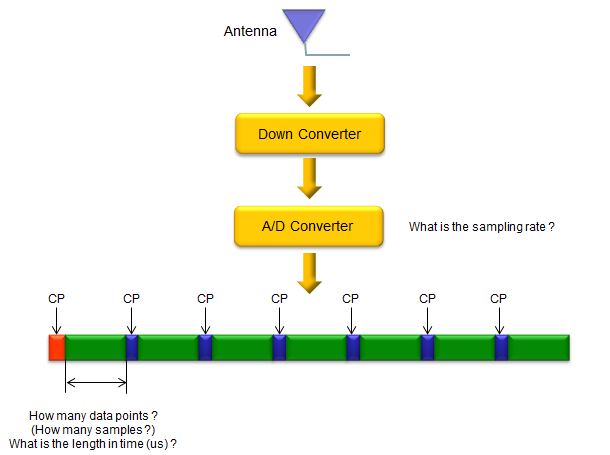Remove CP (Cyclic Prefix) : Now you have the time domain signal and the next step is to remove the Cyclic Prefix. To do this, you have to know exactly what is the number of samples in each symbol and how many samples are assigned for the Cyclic prefix. It depends on the system bandwidth and refer to Physical Layer Parameters - FDD, Downlink for the details.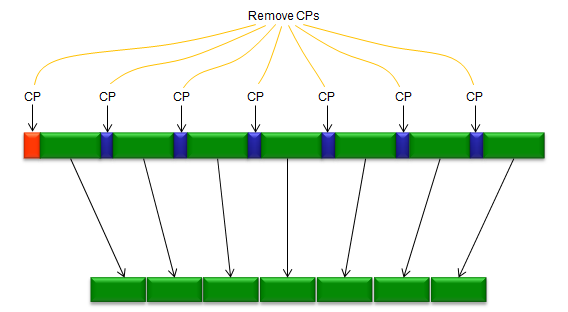Do FFT : Next step is to do FFT for each OFDMA Symbol as shown below. Note that the result of this FFT will include the frequency area which is out side of the resource map. As in other steps, it is important to know how many samples (data points) you will get in the result of FFT and you can get the detailed information on this from Physical Layer Parameters - FDD, Downlink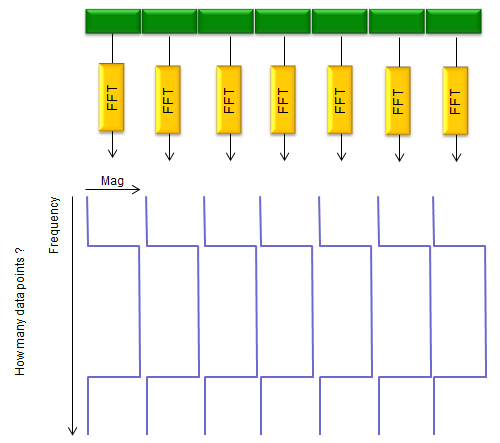Remove the Rejection Area and Map to RE Map : The last step is relatively simple. Just remove the data points which is outside of the sub carriers of the system bandwidth and fill out the resource map with the remaining data. The important things is to cut out the exact data points.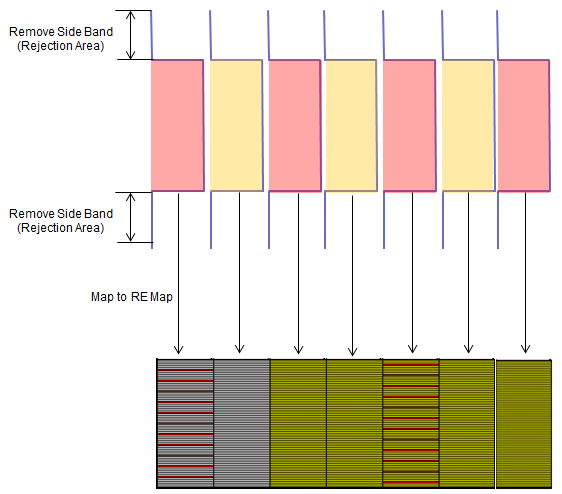In case of Uplink, the term 'RE Map' may mislead or confuse you a lot. Because most of us (including me) are more familiar with downlink frame structure and RE Map than the Uplink, when you talk about 'one resource element', you may easily associate it with 'one 15 Khz subcarrier', but in uplink you should not correlate the one resource element into 15 Khz subcarrier.  As in the downlink case, RE Map refers to the signal structure at the output of [Resource Element Mapper] as shown below. In case of downlink, the data represented in (A) is almost directly trasnsfered to the corresponding to resource element in RE Map (assuming that this is single antenna configuration). and if you take out a data from all the resource elements from RE map and plot it onto a complex plane, you would see a distict constellation, but in case of uplink you would not see such a distict constellation if you take out all the data from resource Map and plot it because the data in each of the resource elements are the result of FFT of the original modulation data.  The constellation would be something as in (D). If you want to get the distict constellation from the data in the UL RE Map, you have to do IFFT (you can see an example in Matlab :ToolBox : LTE : Uplink : PUSCH ).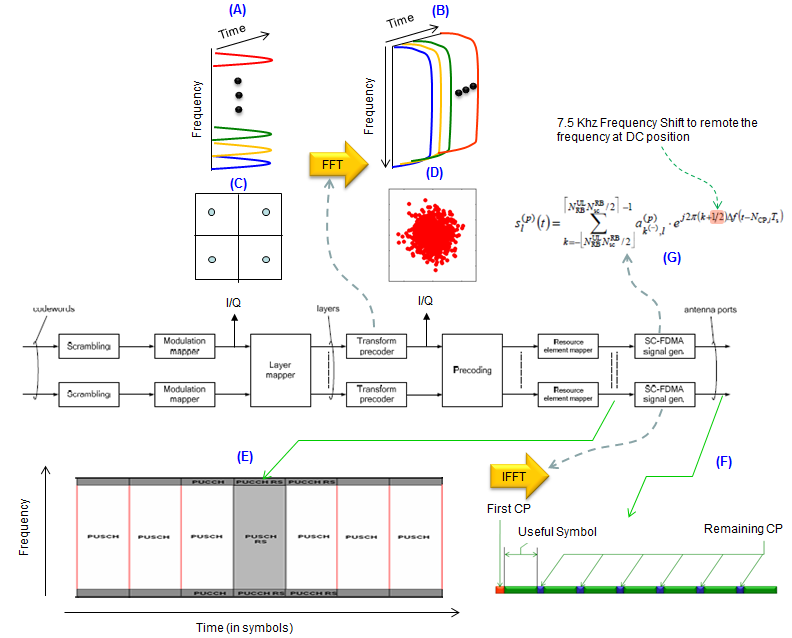If you are interesting in implementing this process in your code (matlab, C etc), I think following illustration would be more helpful. For simplicity, I assumed the case of single antenna (SISO) so that we don't have to care about layer mapping, precoding. Also, I illustrated the case of processing only one symbol. Note that this process applies only for PUSCH. PUSCH-DMRS is a little bit different. Actually, PUSCH-DMRS process is a little bit simpler because it does not go through Transform Precoder.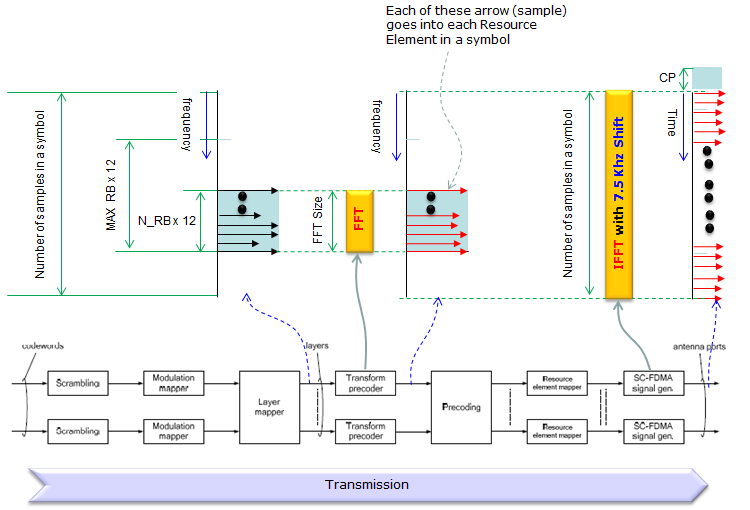This is about receiving the SC-FDMA signal and demodulate it and construct I/Q Constellation (Actually one step further than RE Map) from the demodulated bit stream. In reality, demodulation process is much more complicated than the modulation because you would require a lot of channel estimation and compenstion (Equalization), and finding synchronization. However, I assume that we are in a ideal channel and no need for this kind of compensation or synchronization. Once you understand the overall logic of this process, try your understanding with a real example : LTE UL(SC-FDM) Demodulation.   If you are interesting in implementing this process in your code (matlab, C etc), I think following illustration would be more helpful. For simplicity, I assumed the case of single antenna (SISO) so that we don't have to care about layer mapping, precoding. Also, I illustrated the case of processing only one symbol. Note that this process applies only for PUSCH. PUSCH-DMRS is a little bit different. Actually, PUSCH-DMRS process is a little bit simpler because it does not go through Transform Precoder. Look into the matlab code linked above and try to correlate the code with this illustration.